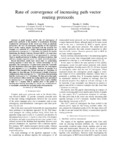## Rate of Convergence of Increasing Path-Vector Routing Protocols

Daggitt, ML
Griffin, TG
##### DOI##### Type
Conference Object
##### Abstract

A good measure of the rate of convergence of path-vector protocols is the number of synchronous iterations required for convergence in the worst case. From an algebraic perspective, the rate of convergence depends on the expressive power of the routing algebra associated with the protocol. For example in a network of n nodes, shortest-path protocols are guaranteed to converge in O(n) iterations. In contrast the algebra underlying the Border Gateway Protocol (BGP) is in some sense too expressive and the protocol is not guaranteed to converge. There is significant interest in finding well-behaved algebras that still have enough expressive power to satisfy network operators.

Recent theoretical results have shown that by constraining routing algebras to those that are strictly increasing'' we can guarantee the convergence of path-vector protocols. Currently the best theoretical worst-case upper bound for the convergence of such algebras is $O(n!)$ iterations. However in practice it is difficult to find examples that do not converge in $n$ iterations. In this paper we close this gap. We first present a family of network configurations that converges in $\Theta(n^2)$ iterations, demonstrating that the worst case is $\Omega(n^2)$ iterations. We then prove that path-vector protocols with a strictly increasing algebra are guaranteed to converge in $O(n^2)$ iterations. Together these results establish a tight $\Theta(n^2)$ bound. This is another piece of the puzzle in showing that strictly increasing" is, at least on a technical level, a reasonable constraint for practical policy-rich protocols. {In memory of Abha Ahuja.

2018-09
2018-07-12
##### Keywords
4904 Pure Mathematics, 49 Mathematical Sciences
##### Journal Title
Proceedings - International Conference on Network Protocols, ICNP
1092-1648
2018-September
IEEE